# Test : Perimeter And Area - 2

## 10 Questions MCQ Test Mathematics for Class 5 (V) - CBSE and NCERT Curriculum | Test : Perimeter And Area - 2

Description
This mock test of Test : Perimeter And Area - 2 for Class 5 helps you for every Class 5 entrance exam. This contains 10 Multiple Choice Questions for Class 5 Test : Perimeter And Area - 2 (mcq) to study with solutions a complete question bank. The solved questions answers in this Test : Perimeter And Area - 2 quiz give you a good mix of easy questions and tough questions. Class 5 students definitely take this Test : Perimeter And Area - 2 exercise for a better result in the exam. You can find other Test : Perimeter And Area - 2 extra questions, long questions & short questions for Class 5 on EduRev as well by searching above.
QUESTION: 1

### ​Find the perimeter of the given figure.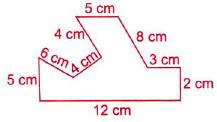Solution:

Perimeter of the given figure
=(5+ 6 + 4 + 4 +5+8+3+ 2+12)cm
= 49 cm

QUESTION: 2

### Find the total area of the shaded parts of the rectangle.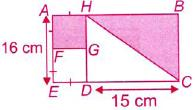Solution:

We have,
AF = FE = ED = GD = GF = HG = AH
Now,  AF + FE= 16 cm
AF + AF= 16 cm
AF = 8 cm
Area of square AFGH = 8 ´ 8 = 64 cm2
Now, area of rectangle HBCD = (15x 16)cm2
= 240 cm2
Area of shaded part of rectangle
= 240/2 = 120 cm
Total shaded area = (64+ 120) cm2
=184 cm2

QUESTION: 3

### ​The given figure is made up of two rectangles Find its total area.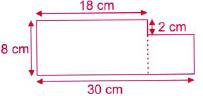Solution:

Area of rectangle ABCD = (18x8) cm2
=144 cm2
Area of rectangle GEFC = (12x6) cm2
= 72 cm2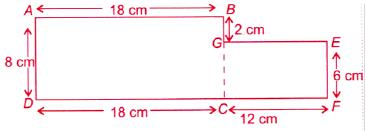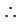Total area = (144 + 72) cm2 = 216 cm2

QUESTION: 4

Find the area of the shaded figure.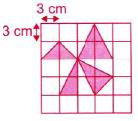Solution:

Area of 1 small square = 3x3 = 9 cm2

Number of shaded squares =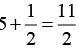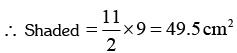QUESTION: 5

​Which shape has the largest area?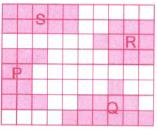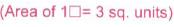Solution:

Area of 1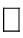= 3 sq. units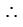Area of shape P = 10 sq. units
Area of shape Q = 11 sq. units.
Area of shape R = 10 sq. units.
Area of shape S = 10 sq. units.
So, shape Q has largest area

QUESTION: 6

​ABCD is a square of perimeter 56 m. Two triangular corners have been cut away as shown in figure. What is the perimeter of the remaining figure?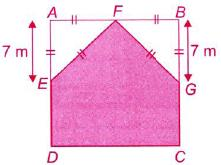Solution:

Perimeter of square = 56 m
4 x side of square = 56 m
=> Side of square = (56/4) = 14 m
Now, AE + ED= 14 m
7 m + ED= 14 m
ED = 7 m
So, AE = AF = £F = FB = BG = FG = 7 m and ED = CG = 7 m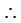Perimeter of remaining figure
= ED + DC + CG + GF + FE
= (7 +14+ 7 + 7 + 7)m = 42m

QUESTION: 7

In the given figure. PQRS is a square and TUVW is a rectangle.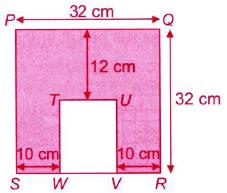Find the remaining area if TUVW was removed from the figure.

Solution:

Area of square PQRS = (32x32) sq. cm =1024 sq. cm
Length of rectangle TUVW = (32- 12) cm
= 20 cm

Breadth of rectangle TUVW = (32- 20) cm =12 cm
So, area of rectangle TUVW
= (12x20) sq. cm
= 240 sq. cm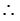Area of remaining figure
= (1024 - 240) sq. cm
= 784 sq.cm

QUESTION: 8

​The figure below is made up of 6 similar squares and 2 triangles. The perimeter of each square is 32 cm. What is the perimeter of the figure?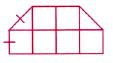Solution:

Perimeter of each square = 32 cm
4xside of square = 32 cm
Side of square = (32/4) cm = 8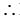Perimeter of the figure = 10x8 = 80 cm

QUESTION: 9

|Find the area of the shaded part in the given figure.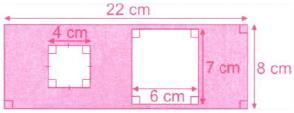Solution:

Area of larger rectangle = (22x8) sq. cm = 176 sq. cm
Area of unshaded rectangle = (7x6) sq. cm
= 42 sq. cm
Area of square = (4 ´ 4) sq. cm = 16 sq. cm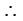Area of shaded region
= 176 sq. cm - (42 + 16) sq. cm
=118 sq. cm

QUESTION: 10

The shaded part in the given figure is covered with cement. If it costs Rs 84 to cement an area of 3 cm2 , find the total cost of cementing.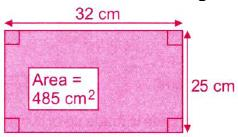Solution:

Area of larger rectangle
= (32x25) cm2 = 800cm2
Also, area of smaller rectangle = 485 cm2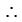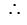Cost of cementing of 1 cm2Cost of cementing of 315 cm2